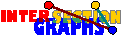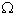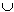# Recognition and Reconstruction

There are two fairly natural problems that arise for every intersection model. Let H be any hypergraph class, and let G(H) denote the class of intersection graphs of members of H.

• Recognition: Given a graph G, decide whether GG(H).
• Reconstruction: Given a graph GG(H), find some or all HH such that G=(H).

The second problem occurs quite naturally in situation where we only get the intersection information, but are interested in the original information. For instance, in a variant of the cafeteria example, where nobody comes twice, one might be interested in who came first. Another natural example is the following:
 Example: Assume there are certain species in a phylogenetic tree. The tree is unknown and has to be reconstructed. What is known are 8 features and which of the species obey which of them. Furthermore, we may make the assumption that each of the features touches a connected part of the tree. We model our information by the intersection multigraph of the species-set of every featureThere are even some cases where we are interested in the model only since optimization of some parameter is doable in the model, but not in the graph alone.

Let me say a few words about the reverse problem, where a class G of graphs is given, and we ask for which hypergraphs H of H the intersection graph lies in G. In general, if the hypergraph is given explicitely, we can compute its intersection graph in polynomial time. Then problems of this kind are algorithmically trivial (if we are only interested in polynomial-time algorithms, not in quickest ones). However, sometimes the hypergraphs are only given implicitely, for instance when the clique hypergraph of a graph is given by the graph itself, or if we represent disks or boxes in d-dimensional space. Then computing the intersection graph may be hard, as it is really the case for clique graphs.

### Characterization and Recognition via duality

For several classes of intersection graphs, recognition algorithms are available. Examples are interval graphs, chordal graphs, cocomparability graphs, line graphs. The maybe most natural approach for characterizing and recognizing graphs of some class of intersection graphs uses duality. Since it has already been used by Krausz, such characterizations are usually called `Krausz-type'. The key idea is to divide the recognition problem into two separate problems which should be solved separately, but the second after the first.

(1) The first subproblem is to find out all star graphs. Obviously, we cannot check all covers of the edge set by complete subgraphs, since their number may be exponential.

(2) If we have all star graphs, then we have H*, and therefore also H. At first sight you may think that we are done, at least for Scheinerman classes, since all we have to check is whether the hyperedges obey property P. But the hypergraph is only given in an abstract form, without labeling of its points. The question remains whether or not it is possible to label the points in such a way that all hyperedges obey our property P.

If subproblems (1) and (2) can be solved efficiently, then we can recognize the corresponding intersection graphs. We shall see that subproblem (1), the problem of identifying the star graphs, is solvable in cases where the hypergraph obeys the so-called Helly-property, or at least does not fail too much. The second subproblem, the layout problem, is often easy in discrete models, but rather difficult in geometric models.

Krausz-type characterizations present characterizations of hypergraphs isomorphic to hypergraphs where all hyperedges obey property P. Or, in the more general case of intersection graphs of hypergraphs from a class H, they present characterizations of the class H. Krausz-type characterizations skip subproblem 1 and only deal with subproblem 2, and even for that, the characterizations given are in some cases not polynomial-time checkable.[K43]: A graph G is a line graph if and only if there is some family of complete subgraphs such that every edge of G lies in exactly one and every vertex of G lies in exactly two of these subgraphs.

Subproblem (1) becomes in most cases trivial if G is triangle-free, since then we have to take essentially all the edges as star graphs. But even for K4-free graphs, the number of possible edge covers by complete graphs may be exponential.

There are two quite natural ways of covering all edges of a graph by complete subgraphs: One is by taking all edges, the other by taking all cliques

That all nontrivial star graphs are edges occurs only if all intersections are `private'--- no three hyperedges have nonempty intersection. This property is rare, but can be achieved, for instance, for intersection of straight lines, or intersections of chords of the cicle. Thus, in the duality approach for recognizing members of G(straight lines) or circle graphs, the first step is easy.

What about cliques? There are few models where all star graphs are cliques, but what about the converse? When are all cliques star graphs? Exactly if,

(H) for every set of pairwise intersecting hyperedges, the total intersection is nonempty.

This is the Helly-property for hypergraphs. It turns out that models where this Helly-property is automatically fulfilled are easier to handle.

### Hard cases

Nevertheless, recognition is hard for most models. Examples are intersection graphs of k-uniform hypergraphs for k > 2, linear k-uniform hypergraphs for k>2, or (k-1)-intersection graphs of k-uniform hypergraphs where k > 2. However, all these graphs can be recognized almost surely.

### Forbidden induced subgraphs

Recall that Scheinerman intersection classes are closed under induced subgraphs. Therefore there is always a characterization by forbidden induced subgraphs. There are only two difficulties with this approach: First, the complete list of these graphs may not be easy to get. Secondly, the list may be infinite. This could be fine, as for chordal graphs, for instance, but it may even be that the list lacks a finite description.

The nicest result in this direction is Beineke's famous characterization of line graphs by a list of nine small forbidden induced subgraphs.

For intersection graphs of 3-linear uniform hypergraphs of high enough minimum degree, there are also characterizations by forbidden induced subgraphs [NRSS82], [MT97].

But in most cases, all one could hope for is a list of some forbidden induced subgraphs. Although these lists do not lead to recognition, some of these forbidden induced subgraphs are rather important, like octahedrons, or graphs Kn -e, for instance, and should be routinely checked when dealing with classes of intersection graphs.

What about minors? By Robertson and Seymour's proof of the Wagner conjecture, every class of graphs closed under minors can be described by a finite list of forbidden minors. Unfortunately, few intersection classes are closed under minors---deleting an edge is not allowed, we cannot make two sets disjoint without affecting the intersections with the other sets. On the other hand, many models behave quite well under contractions. Let G =(A,(Sx/xV)), and let G/yz be the graph obtained by contracting an edge yz. We get a representation of G/yz by letting all sets Sv, with distinct y and z, unchanged and taking SySz for the new vertex. Therefore a Scheinerman class G(P) is closed under edge contraction if SySz has again property P whenever Sx, Sy have property P and have nonempty intersection. Examples of such properties are being intervals of the real line, being subtree of a given tree, or being a connected subset of Rd.

Such classes are closed under induced minors, where we only allow edge contraction and vertex deletion---edge deletion is forbidden. Unfortunately, the induced minor behave much worse than minors. There are classes closed under induced minors which cannot be described by a finite list of forbidden induced minors. Even the problem to test whether a given graph H is an induced minor could be NP-complete, for some fixed H [FKMP95]. Thus being closed under induced minors does not seem to help much.

Back to the start page for intersection graphs.

Erich Prisner